### Discover more content...

Enter some keywords in the search box above, we will do our best to offer you relevant results.

### We're sorry!

We couldn't find any results for your search. Please try again with another keywords.

## 一. Evaluating a Learning Algorithm

• 采集更多的样本
错误的认为样本越多越好，其实数据多并不是越好。

• 降低特征维度
降维可能去掉了有用的特征。

• 采集更多的特征
增加了计算负担，也可能导致过拟合。

• 进行高次多项式回归
过高的多项式可能造成过拟合。

• 调试正规化参数 $\lambda$,增大或者减少 $\lambda$
增大或者减少都是凭感觉。

### 1. Evaluating a Hypothesis 评价假设函数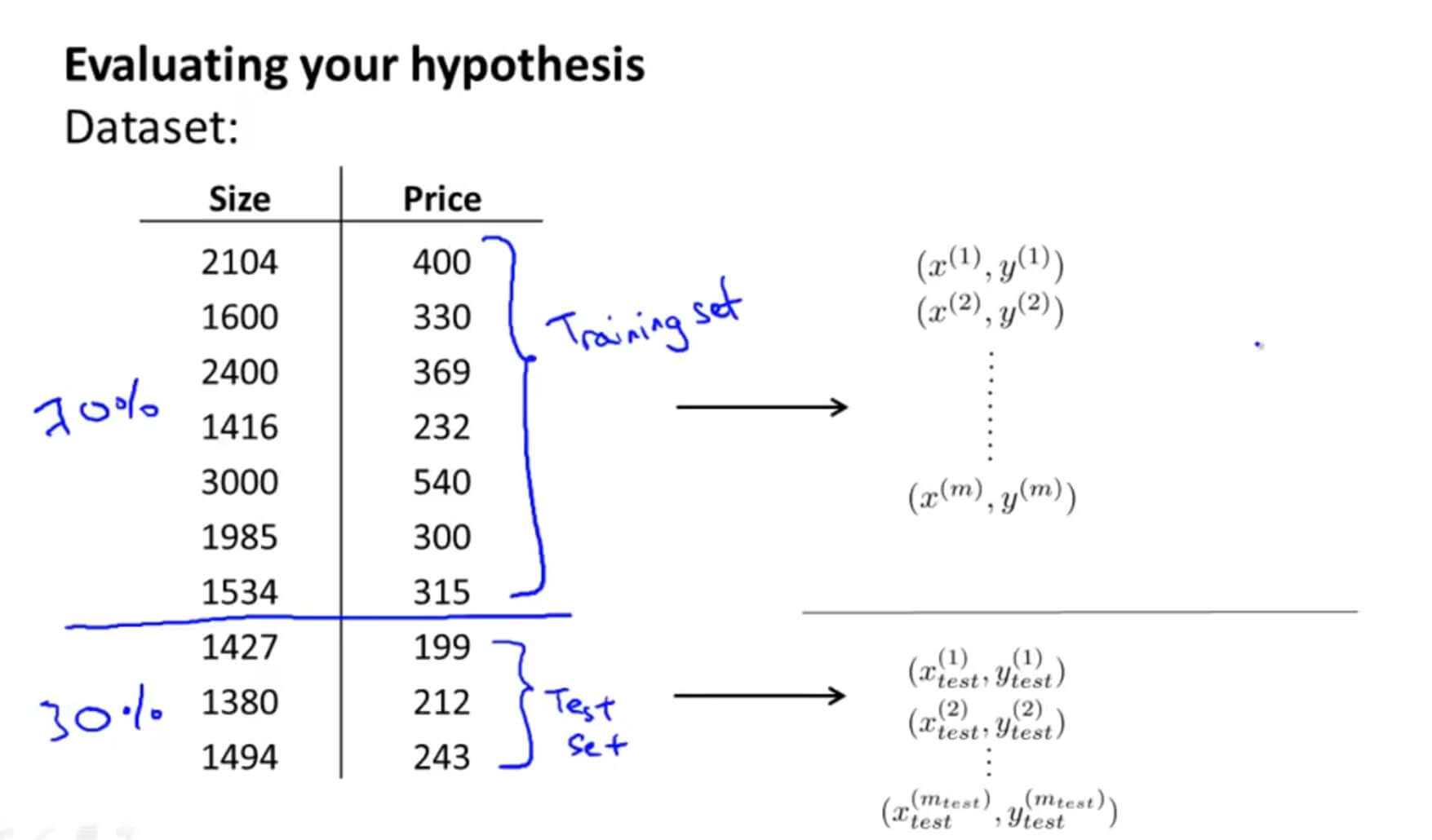1. 对训练集进行学习得到参数 $\Theta$ ，也就是利用训练集最小化训练误差 $J_{train}(\Theta)$
2. 计算出测试误差 $J_{test}(\Theta)$，取出之前从训练集中学习得到的参数 $\Theta$ 放在这里，来计算测试误差。

$$J_{test}(\theta)=\frac{1}{2m_{test}}\sum_{i=1}^{m_{test}}{(h_\theta(x^{(i)}_{test})-y^{(i)}_{test})^2}$$

$$J_{test}(\theta)=-\frac{1}{m_{test}}\sum_{i=1}^{m_{test}}{y^{(i)}_{test}logh_\theta(x^{(i)}_{test})+(1-y^{(i)}_{test})logh_\theta(x^{(i)}_{test})}$$

$$err(h_\theta(x),y)=\left\{\begin{matrix} 1 \;\; ( if \;\;\; h_\theta(x) \geqslant 0.5 , y=0 \;\;\;or\;\;\; if\;\;\; h_\theta(x) < 0.5 , y=1 ) \\ 0 \;\;\;( otherwise ) \;\;\;\;\;\;\;\;\;\;\;\;\;\;\;\;\;\;\;\;\;\;\;\;\;\;\;\;\;\;\;\;\;\;\;\;\;\;\;\;\;\;\;\;\;\;\;\;\;\;\;\;\; \end{matrix}\right.$$

$( if \;\;\; h_\theta(x) \geqslant 0.5 , y=0 \;\;\;or\;\;\; if\;\;\; h_\theta(x) < 0.5 , y=1 )$

$$Test\;Error=\frac{1}{m_{test}}\sum_{i=1}^{m_{test}}err(h_{\theta}(x^{(i)}_{test}),y^{(i)}_{test})$$

### 2. Model Selection and Train/Validation/Test Sets 模型选择和训练集/验证集/测试集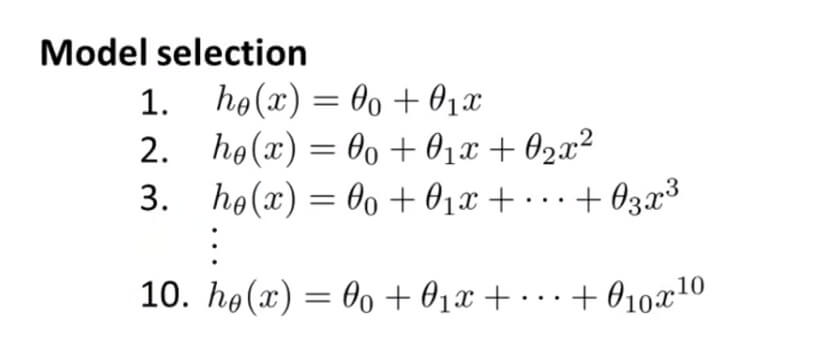$$J_{train}(\theta) = \frac{1}{2m}\sum_{i=1}^{m}(h_\theta(x^{(i)})-y^{(i)})^{2}$$

$$J_{cv}(\theta) = \frac{1}{2m_{cv}}\sum_{i=1}^{m_{cv}}(h_\theta(x^{(i)}_{cv})-y^{(i)}_{cv})^{2}$$

$$J_{test}(\theta) = \frac{1}{2m_{test}}\sum_{i=1}^{m}(h_\theta(x^{(i)}_{test})-y^{(i)}_{test})^{2}$$

1. 利用训练集的数据代入每一个多项式模型。
2. 用交叉验证集的数据找出最小误差的多项式模型。
3. 最后在测试集再找出相对较少误差的那个模型。

## 二. Bias vs. Variance

### 1. Diagnosing Bias vs. Variance 诊断偏差和方差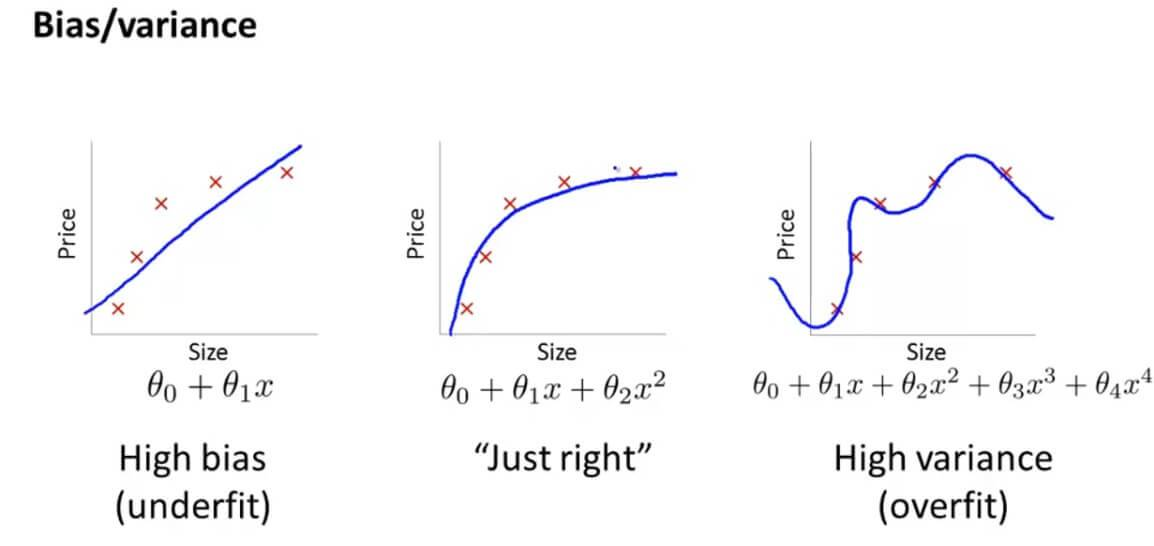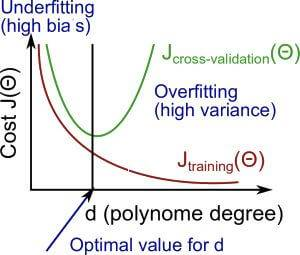$$\left\{\begin{matrix} J_{train}(\theta) \;\;\;is\;\; low\\ J_{cv}(\theta)>>J_{test}(\theta) \end{matrix}\right.$$

$$\left\{\begin{matrix} J_{train}(\theta),J_{cv}(\theta)\;\;\; is \;\; high\\ J_{cv}(\theta) \approx J_{test}(\theta) \end{matrix}\right.$$

### 2. Regularization and Bias/Variance 正则化的偏差和方差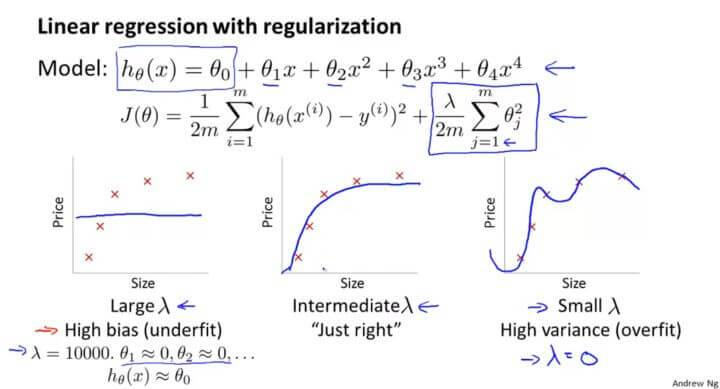$\lambda$的取值不能过大也不能过小。

$\lambda$的取值可以在 $\left[0,0.01,0.02,0.04,0.08,0.16,0.32,0.64,1.28,2.56,5.12,10.24\right]$，在这12个不同的模型中针对每一个 $\lambda$ 的值，都去计算出一个最小代价函数,从而得到 $\Theta^{(i)}$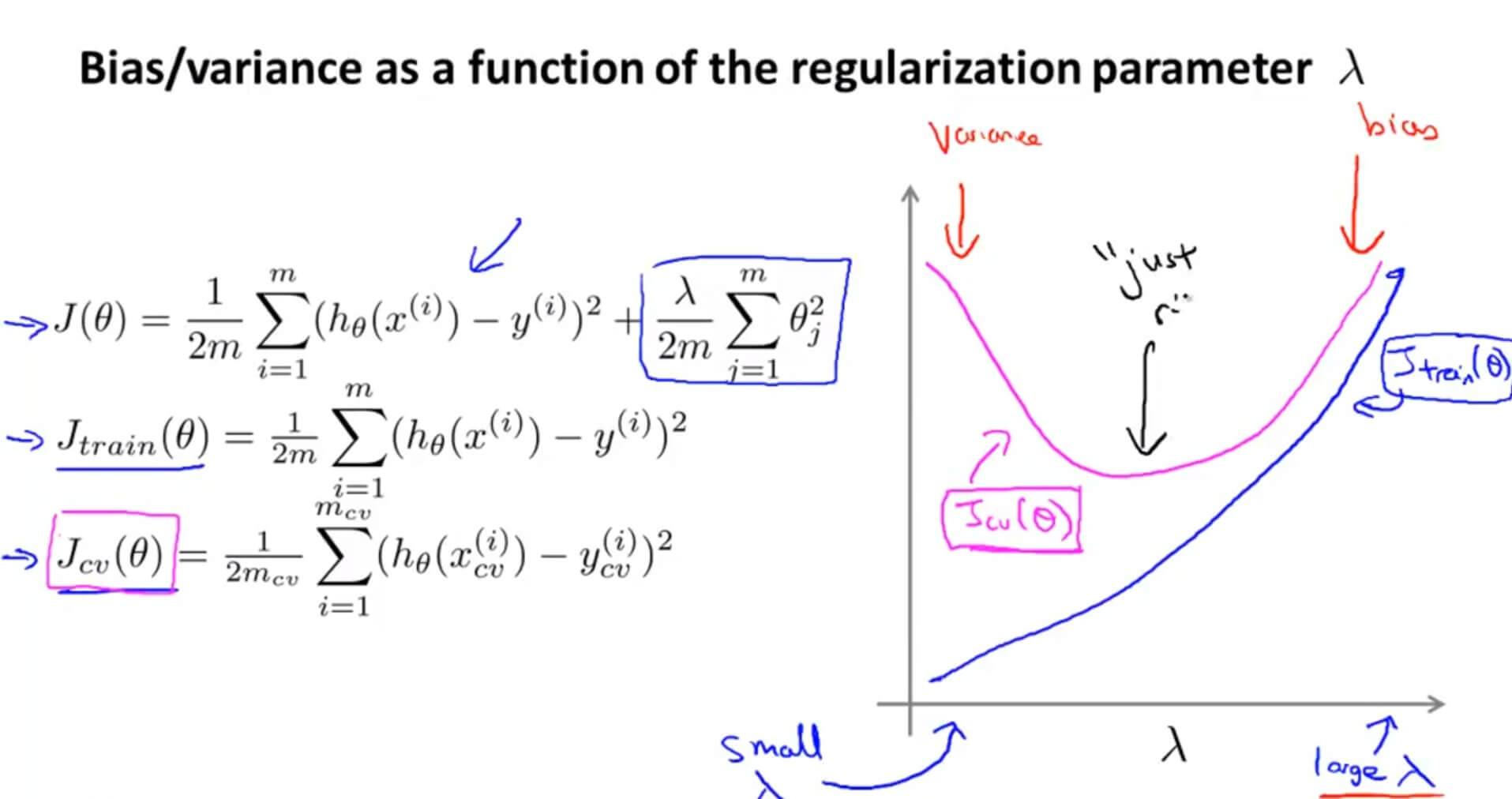### 3. Learning Curves 学习曲线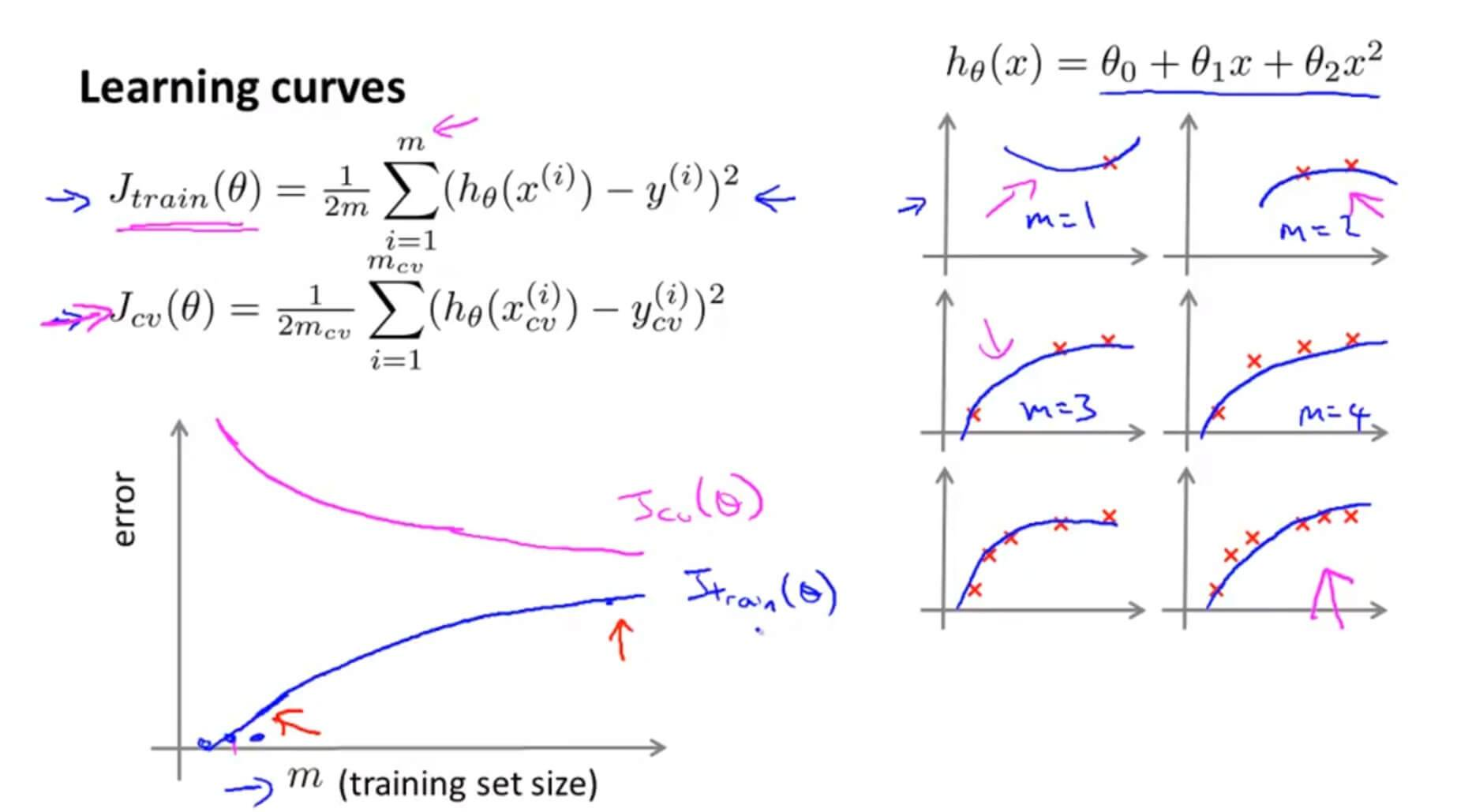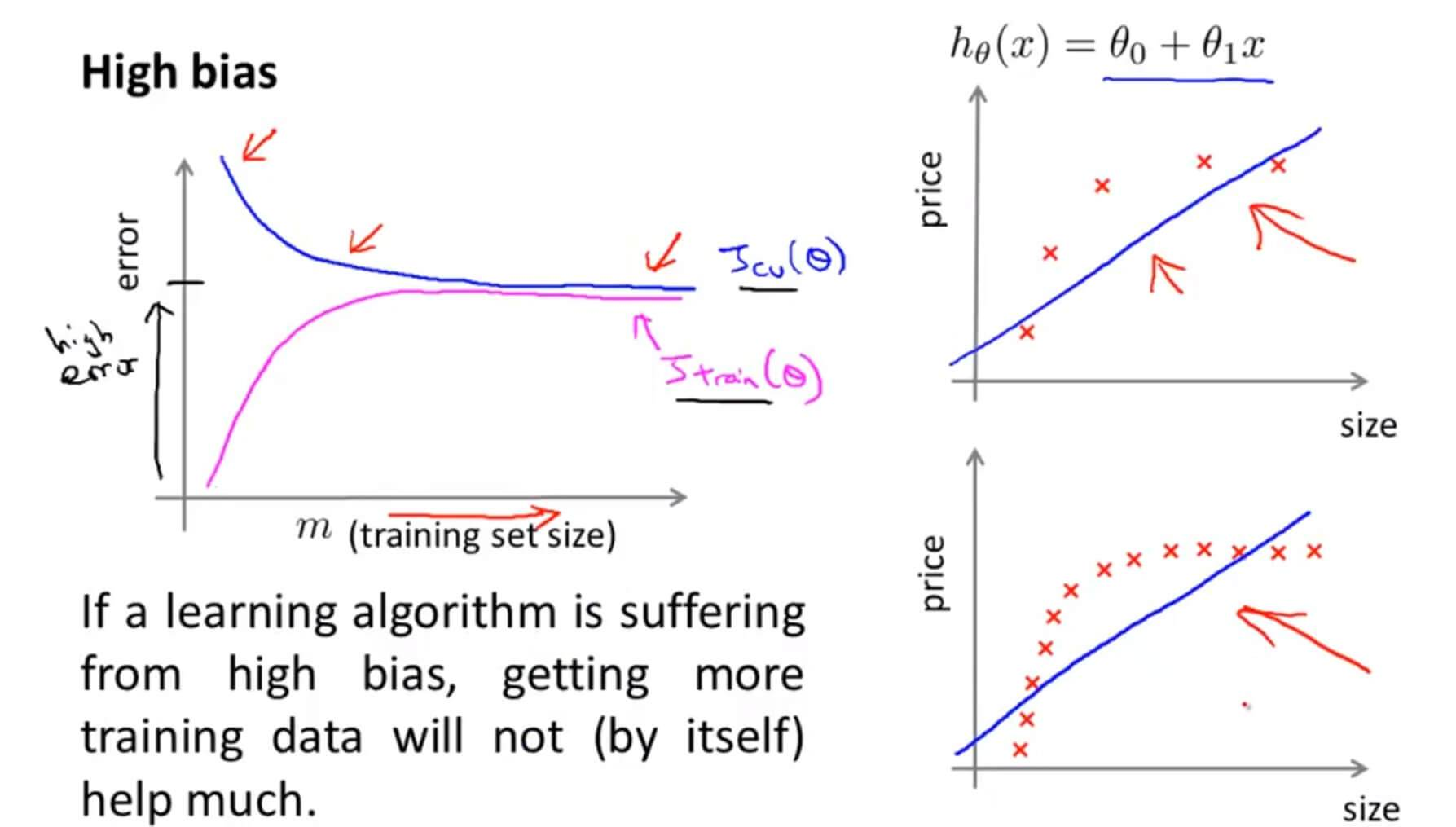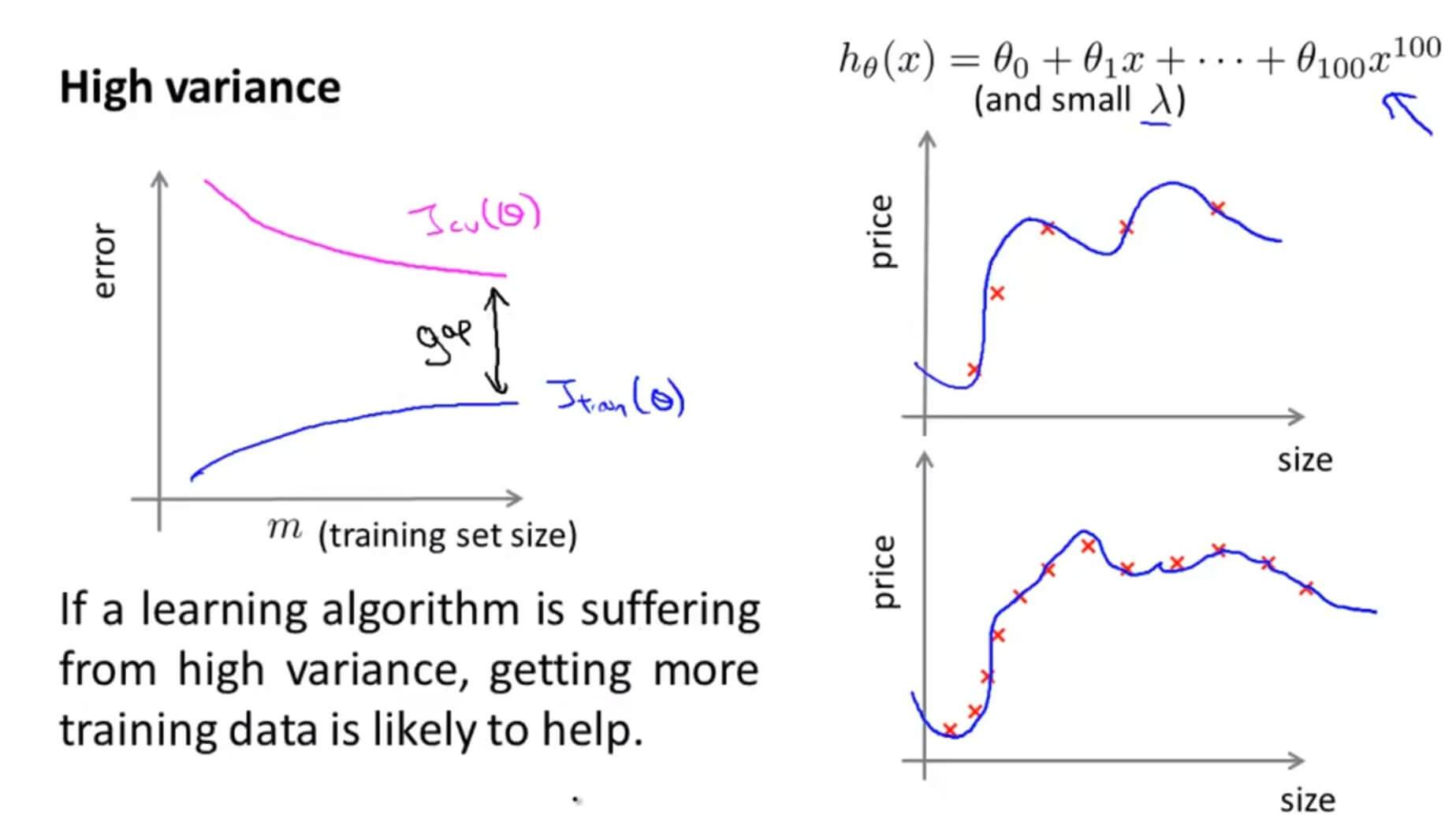### 4. Deciding What to Do Next Revisited 决定下一步该做什么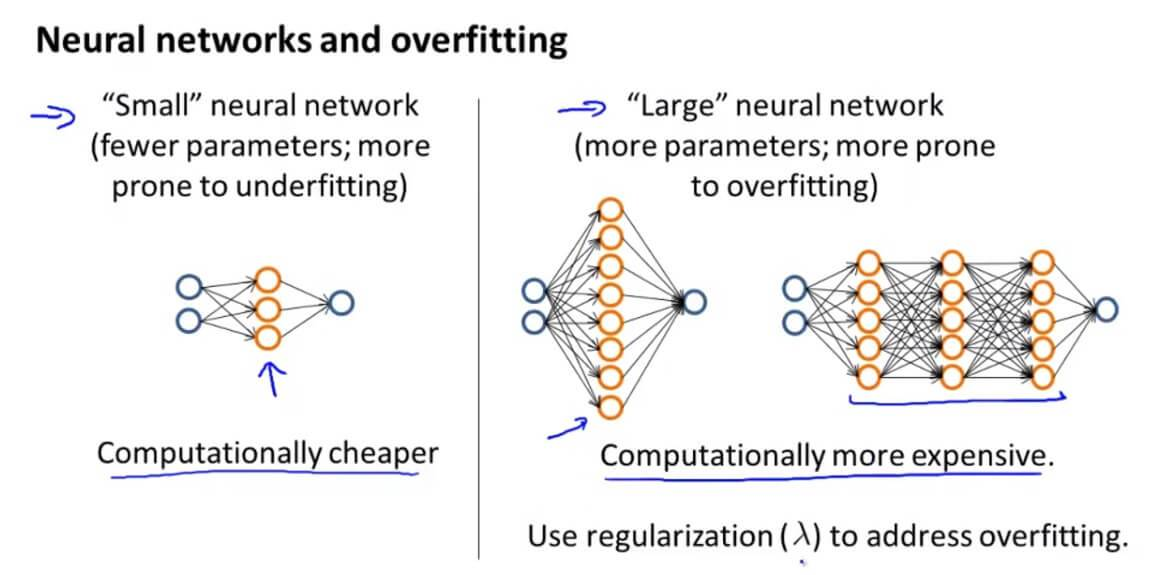• 低阶多项式（低模型复杂度）具有高偏差和低方差。在这种情况下，该模型很难一致
• 高阶多项式（高模型复杂度）非常适合训练数据，测试数据极其糟糕。这些对训练数据的偏倚低，但差异很大
• 实际上，我们希望选择一个介于两者之间的模型，它可以很好地推广，但也可以很好地适合数据。

## 三. Advice for Applying Machine Learning 测试

### 1. Question 1

You train a learning algorithm, and find that it has unacceptably high error on the test set. You plot the learning curve, and obtain the figure below. Is the algorithm suffering from high bias, high variance, or neither?A. High variance

B. Neither

C. High bias

### 2. Question 2

Suppose you have implemented regularized logistic regression to classify what object is in an image (i.e., to do object recognition). However, when you test your hypothesis on a new set of images, you find that it makes unacceptably large errors with its predictions on the new images. However, your hypothesis performs well (has low error) on the training set. Which of the following are promising steps to take? Check all that apply.

A. Try adding polynomial features.

B. Get more training examples.

C. Try using a smaller set of features.

D. Use fewer training examples.

### 3. Question 3

Suppose you have implemented regularized logistic regression to predict what items customers will purchase on a web shopping site. However, when you test your hypothesis on a new set of customers, you find that it makes unacceptably large errors in its predictions. Furthermore, the hypothesis performs poorly on the training set. Which of the following might be promising steps to take? Check all that apply.

A. Try using a smaller set of features.

B. Try adding polynomial features.

C. Try to obtain and use additional features.

D. Try increasing the regularization parameter $\lambda$.

### 4. Question 4

Which of the following statements are true? Check all that apply.

A. Suppose you are training a regularized linear regression model. The recommended way to choose what value of regularization parameter $\lambda$ to use is to choose the value of $\lambda$ which gives the lowest test set error.

B. The performance of a learning algorithm on the training set will typically be better than its performance on the test set.

C. Suppose you are training a regularized linear regression model.The recommended way to choose what value of regularization parameter $\lambda$ to use is to choose the value of $\lambda$ which gives the lowest training set error.

D. Suppose you are training a regularized linear regression model. The recommended way to choose what value of regularization parameter $\lambda$ to use is to choose the value of $\lambda$ which gives the lowest cross validation error.

### 5. Question 5

Which of the following statements are true? Check all that apply.

A. If a learning algorithm is suffering from high variance, adding more training examples is likely to improve the test error.

B. We always prefer models with high variance (over those with high bias) as they will able to better fit the training set.

C. If a learning algorithm is suffering from high bias, only adding more training examples may not improve the test error significantly.

D. When debugging learning algorithms, it is useful to plot a learning curve to understand if there is a high bias or high variance problem.

A 过拟合高方差，增加样本数量有用。
B 高偏差和高方差的模型都不好。
C 增加训练样本对于欠拟合是没用的正确。
D 绘制学习曲线有利于帮助我们分析问题正确。

GitHub Repo：Halfrost-Field

Follow: halfrost · GitHubPrevious Post

Next Post